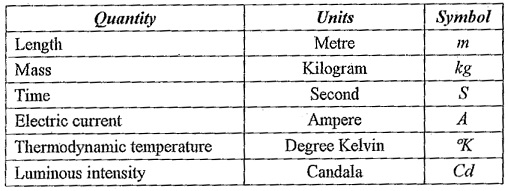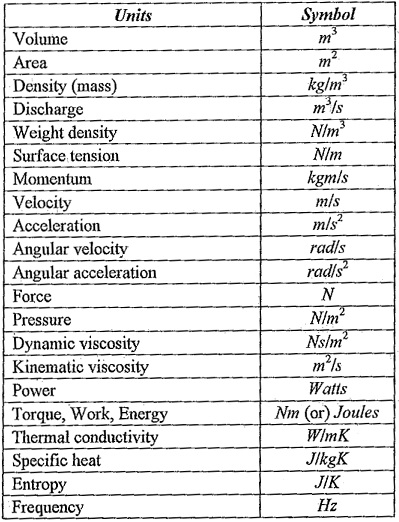## Types of Unit System:

All physical quantities are measured in certain units. There are two Types of Unit System, namely:

1. Fundamental units
2. Derived units

### Fundamental Units:

All the physical quantities arc expressed of the following three fundamental Types of Unit System:

1. Length (L)
2. Mass (M)
3. Time (T)

### Derived Units:

Some units called derived units are expressed in terms of fundamental units, such as units of area velocity, acceleration, pressure etc.

### System of units:

The following are the four Types of Unit System are internationally accepted.

1. C.G.S units
2. F.P.S units
3. M.K.S units
4. S.I. units

CG.S units: In this system the fundamental units of length, mass and time are taken as centimeter, gram, and second respectively.

F.P.S units: In this system the fundamental units of length, mass and time are taken as foot, pound, and second respectively.

M.K.S units: In this system the fundamental units of length, mass and time are taken as meter, kilogram, and second respectively. The M.K.S. units are called as gravitational units or engineer’s units.

S.I. units (International System of Units): This system has six basic units, two supplementary units and twenty-seven derived units.

The six basic Types of Unit System are as follows:There are two supplementary units. One for measure of plane angle called the radian (rad) and other for solid angle called stearadian (Sr) some of the derived units are shown in table below.### Liquid and Gas:

#### Liquid:

It is a fluid which possesses a definite volume. The volume may be vary slightly with change in temperature and pressure. Liquid can be compressed to a small extend. But for all practical purposes, it is regarded as in compressible.

#### Gas:

It is fluid which have a tendency to expand and fill the container in which they are kept. They do not have any free surface. It is compressible fluids. Even a slight change in temperature it has a significant effect on its volume and pressure. Some of the examples of fluids are water, air, hydrogen gas, honey, oil, paint, glycerin, blood etc.

Fluid mechanics is the branch of science which deals with the behaviour of a fluid at rest as well as in motion. The behaviour of a fluid when it is at rest is called ‘Fluid statics‘. The behaviour of a fluid when it is in motion without considering pressure force is called as ‘Fluid kinematics‘. The behaviour of a fluid when it is in motion with considering pressure force is called as ‘Fluid dynamics‘.

Scroll to Top